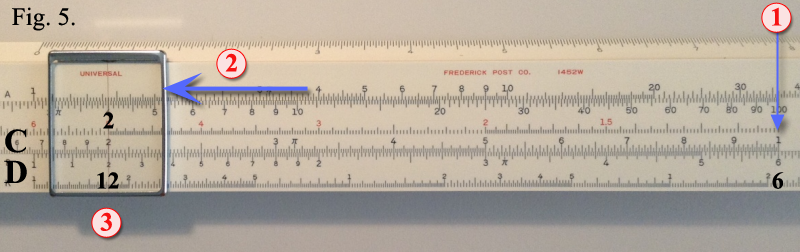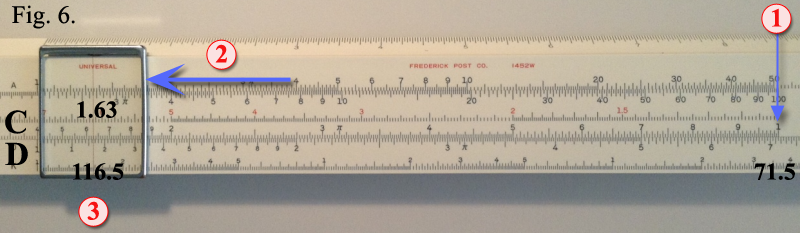Reading the ScalesThe C and D scales are most commonly used for multiplication and division. These scales have identical graduations and appear on all slide rules.Their left and right-hand ends marked with the numeral ‘‘1’’, and are called left index and right index, respectively. The major divisions are marked from 2 through 9. Each major division is further divided into tenths. More complete divisions are given for values between 1 and 2, covering the left-hand end of the scale. This section is further sub-divided into ten major sub-divisions from 1 to 9, representing the values 11 to 19 (on the POST four inch rule, only 15 mark is numbered, due to lack of space). The tenths are still further sub-divided into tenths, fifths or halves, as space permits.On the previous page, you will find that Figure 1 (10 inch rule) illustrate how to read various values on a typical slide rule scale. Familiarize yourself with the scale so you will know how to find these examples.

 First Digit is GuideNote that all numbers that begin with the numeral 1, regardless of the position of the decimal point, are found somewhere between the left index and the major division 2. This space covers approximately the left-hand third of the entire scale. All numbers beginning in 2 are found between the major divisions 2 and 3, numbers beginning in 3 between major divisions 3 and 4, and so on.When it is necessary to read a scale at a point between two graduations, the setting must be estimated to the nearest fraction of a division.All numbers that have the same digit value (e.g. 0.00274, 2.74, or 27,400) are found at the same point on the slide rule scale, regardless of the position of the decimal point. Example shown in Figure 2.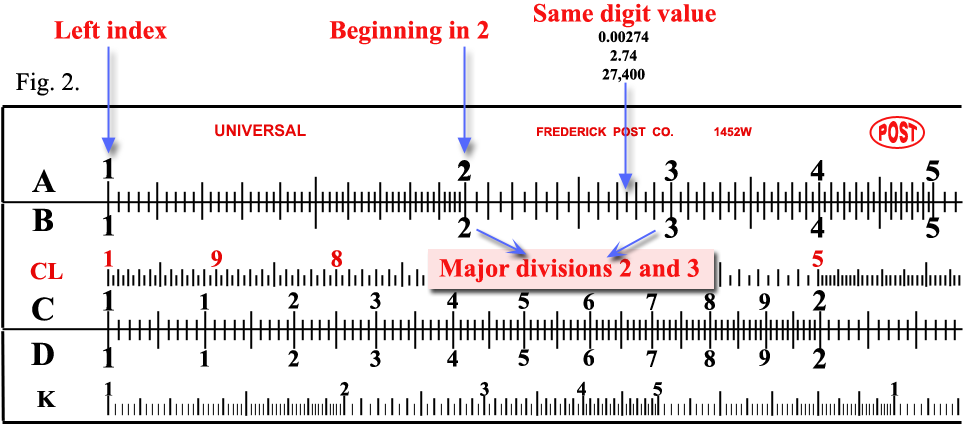MULTIPLICATIONTo illustrate a typical slide rule procedure, solve the following simple problem as shown in Figure 3: 2 × 3 = 6 Set the left index of the C over 2 on D. Set hairline over 3 on C and find the slide rule answer 6 on D.The slide rule solves more complex problems with equal ease. For example: 1960 × 3.25 = 6370, as shown in Figure 4.Set the left index of the C over 196 on D. Set hairline over 325 on C and find the slide rule answer 637 on D.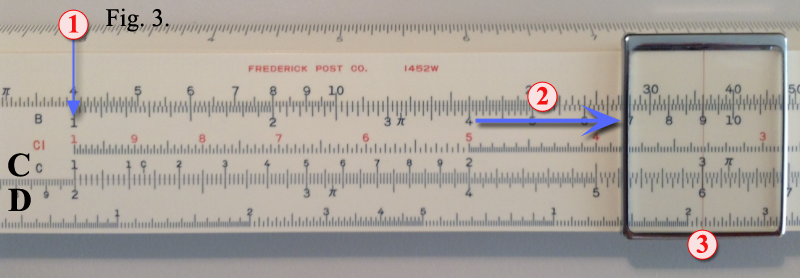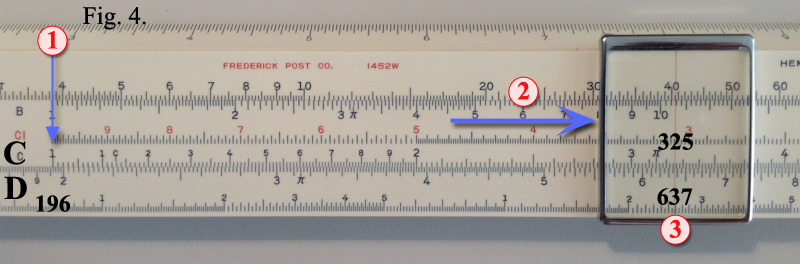Decimal PointsIn the above example, the location of the decimal point can be determined by a quick mental calculation using round figures close to the numbers in the problem. Thus: 2000 × 3 = 6000. Our answer, therefore, must be 4 digits to the left of the decimal point, or 6370. Use of Right IndexIf the values in a problem are such that the multiplier on the C scale extends out beyond the limits of the D scale, then use the right index of C instead of the left index. See figure 5 for an illustration of this example: 6 × 2 = 12If we set the left index of C over 6 on the D scale, we find that 2 on the C scale goes beyond the right-hand end of the D scale. So we proceed as follows: Set the right index of the C scale over 6 on the D scale. Under 2 on the C scale, we now find the answer 12 on the D scale.Here is another example: 71.5 × 1.63 = 116.5. Set the right index of the C over 715 on the D scale. Set the cursor hairline over 163 on the C scale and read the slide rule answer 1165 under the hairline on the D scale. See figure 6.The decimal point is located by making a quick mental calculation (70 × 1.5 = 105.0) Thus the answer in this example is 116.5.